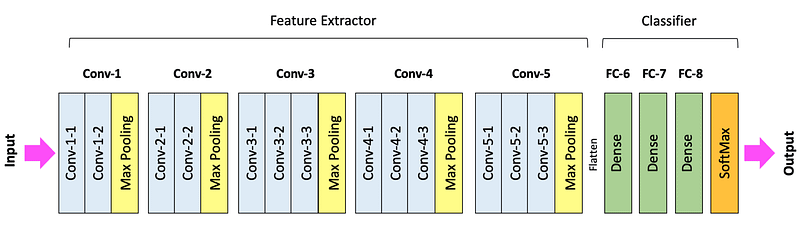# Delving into Deep Learning: An Exhaustive Guide to Understanding and Implementing Convolutional Neural Networks### Introduction

Convolutional Neural Networks (CNNs) are a class of deep neural networks widely used in the field of computer vision, becoming the state-of-the-art solution for various tasks, including image and video recognition, recommender systems, and natural language processing. CNNs are designed to automatically and adaptively learn spatial hierarchies of features from tasks with input data in 2D grids. In this comprehensive guide, we’ll unravel the complex structure of CNNs and delve into their inner workings.

### Basics of Convolutional Neural Networks

A Convolutional Neural Network is a type of deep learning algorithm which can take in an input image, assign importance to various aspects in the image, and be able to differentiate one from the other. The pre-processing required in a ConvNet is much lower as compared to other classification algorithms.

CNNs are composed of several layers through which input data is passed and transformed into output data, i.e., the result of the neural network’s “learning.” The types of layers in a CNN typically include:

Input Layer: The input layer is the first layer in the network where the raw pixel data from the image is fed into the network.

Convolutional Layer: The convolutional layer performs a mathematical operation called a “convolution” on the input data. This operation involves sliding a filter or a “kernel” across the input image and computing the dot product between the weights of the filter and the input image.

ReLU (Rectified Linear Unit) Layer: The ReLU layer applies the non-linear function max(0,x) element-wise to the input data. This operation introduces non-linear properties to the model, allowing it to learn more complex patterns.

Pooling Layer: The pooling layer, often also known as a subsampling layer, reduces the spatial dimensions (width and height) of the input volume. This layer helps decrease the computational complexity, memory usage, and number of parameters.

Fully Connected Layer: The fully connected layer connects every neuron in one layer to every neuron in another layer, which is why it’s called “fully connected.” It is the last layer in a CNN and is used for classifying the images.

### CNN Architecture

The fundamental CNN architecture consists of a stack of Convolutional Layers, followed by ReLU layers, Pooling layers, and fully connected layers. The input to the CNN is a 2-dimensional array of pixels, which is passed through the stack of layers, resulting in an output that corresponds to the class labels of the image.

#### 1. Convolutional Layer

In the Convolutional Layer, various filters are applied to the input image, and feature maps are generated. These filters help in feature detection, such as edges, curves, etc., in the image.

#### 2. ReLU Layer

The Rectified Linear Unit (ReLU) layer applies the non-linear function max(0,x) element-wise to the input data. This operation introduces non-linear properties to the model, allowing it to learn and adapt more complex patterns.

#### 3. Pooling Layer

The Pooling layer, also known as a subsampling layer, reduces the spatial size of the feature map, leading to a decrease in the computational complexity, memory usage, and number of parameters. It can perform different functions like Max Pooling, Average Pooling, etc.

#### 4. Fully Connected Layer

The Fully Connected layer connects every neuron in one layer to every neuron in another layer. It takes an input from all feature maps and generates the final output of the network. The output could be a single unit for binary classification tasks, or multiple units for multi-class tasks.

### Understanding Convolution

The “convolution” in “convolutional neural networks” refers to the mathematical operation that is performed on the input data in the network’s convolutional layers. In the context of a CNN, a convolution is a linear operation that involves the multiplication of a set of weights with the input, much like traditional neural networks. However, unlike fully connected networks, which use the same weights for every input, CNNs have unique weights in their convolutions.

### Conclusion

Convolutional Neural Networks have revolutionized the field of computer vision by providing state-of-the-art solutions to many complex tasks. Their unique architecture, specifically designed to process 2D data, makes them exceptionally good at image and video recognition tasks. By understanding the fundamentals of CNNs, data scientists and AI practitioners can harness their power and use them to build highly effective and efficient models for a wide range of tasks. This detailed guide is just the beginning of your journey into the fascinating world of convolutional neural networks.

# Personal Career & Learning Guide for Data Analyst, Data Engineer and Data Scientist

## Applied Machine Learning & Data Science Projects and Coding Recipes for Beginners

A list of FREE programming examples together with eTutorials & eBooks @ SETScholars

# Projects and Coding Recipes, eTutorials and eBooks: The best All-in-One resources for Data Analyst, Data Scientist, Machine Learning Engineer and Software Developer

Topics included:Classification, Clustering, Regression, Forecasting, Algorithms, Data Structures, Data Analytics & Data Science, Deep Learning, Machine Learning, Programming Languages and Software Tools & Packages.
(Discount is valid for limited time only)## Find more … …

Learn Keras by Example – How to Build Convolutional Neural Network

Deep Learning in R with Dropout Layer | Data Science for Beginners | Regression | Tensorflow | Keras

How to connect MYSQL Database in Python